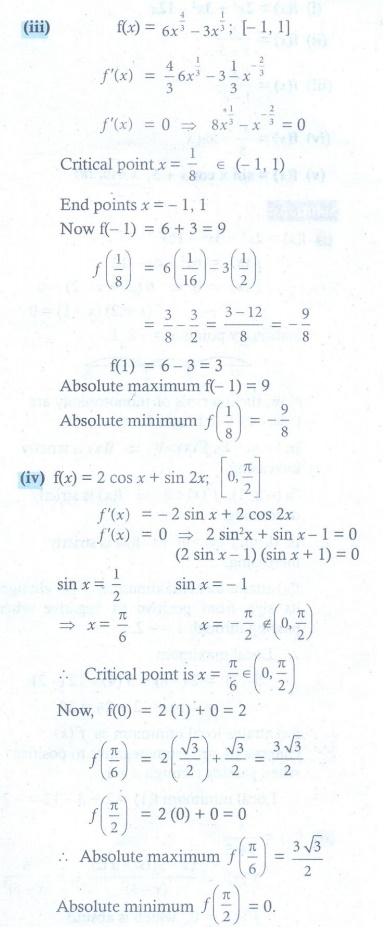Home | | Maths 12th Std | Exercise 7.6 : Applications of First Derivative

# Exercise 7.6 : Applications of First Derivative

Maths Book back answers and solution for Exercise questions - Mathematics : Applications of Differential Calculus: Applications of First Derivative: Problem Questions with Answer, Solution

EXERCISE 7.6

1. Find the absolute extrema of the following functions on the given closed interval.

(i) ( x ) = x 2 ŌłÆ12+10 ; [1, 2]

(i) ( x ) = 3x4 ŌłÆ 4x3 ; [ŌłÆ1, 2]

(i) f (x) = 6x4/3 ŌłÆ3x1/3 ; [ŌłÆ1, 1]

(iv) f (x) = 2 cos x + sin 2x ; [0, ŽĆ/2]2. Find the intervals of monotonicities and hence find the local extremum for the following functions:

(i) f (x) = 2x3 + 3x2 ŌłÆ12x ŌĆā

(ii) f (x) = x x ŌłÆ 5

(iii) f (x) = ex / 1-x3

(iv) f (x) = ex/3 ŌłÆ log x

(v) f (x) = sin x cos x + 5, x Ōłł(0, 2ŽĆ )(1)

(i) absolute maximum = ŌłÆ1 , absolute minimum = ŌłÆ26

(ii) absolute maximum = 16 , absolute minimum = ŌłÆ1

(iii) absolute maximum = 9 , absolute minimum = ŌłÆ 9/8

(iv) absolute maximum = 3ŌłÜ3 / 2 , absolute minimum = 0

(2)

(i) strictly increasing on ( -Ōł×, 2 ) and (1,Ōł×) , strictly decreasing on (ŌłÆ2,1)

local maximum = 20

local minimum = ŌłÆ7

(ii) strictly decreasing on ( ŌłÆŌł×, 5) and (5, Ōł×) . No local extremum.

(iii) strictly increasing on ( ŌłÆŌł×, Ōł×). No local extremum.

(iv) strictly decreasing on ( 0,1) , strictly increasing on (1, Ōł×). local minimum = 1/3

(v) strictly increasing onstrictly decreasing on ,. local maximum= 11/2 at x = ŽĆ / 4, 5ŽĆ /4.

local minimum= 9/2 at x = 3ŽĆ / 4, 7ŽĆ / 4.

Tags : Problem Questions with Answer, Solution , 12th Maths : UNIT 7 : Applications of Differential Calculus
Study Material, Lecturing Notes, Assignment, Reference, Wiki description explanation, brief detail
12th Maths : UNIT 7 : Applications of Differential Calculus : Exercise 7.6 : Applications of First Derivative | Problem Questions with Answer, Solution